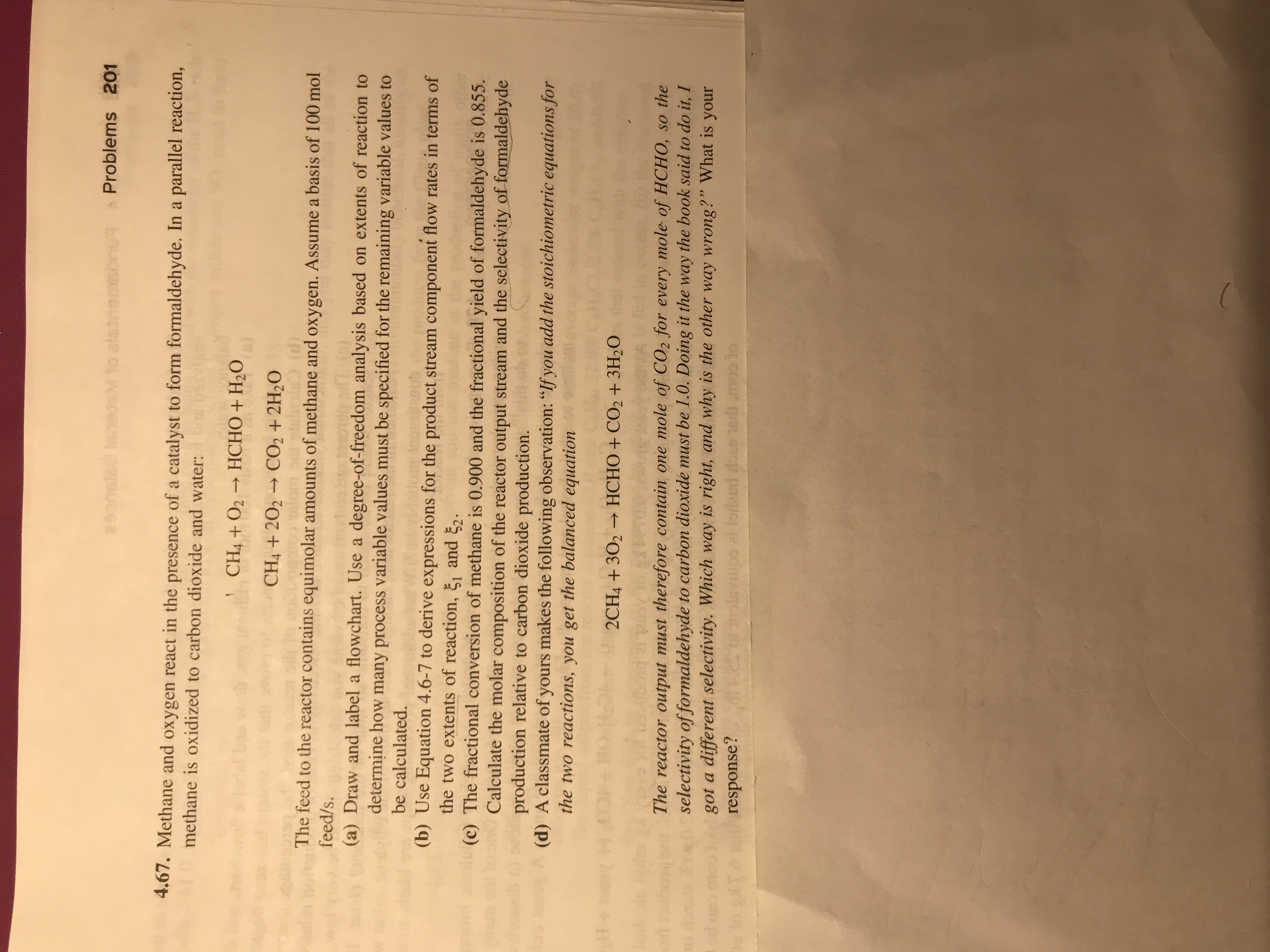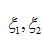# Problems 2014,67. Methane and oxygen react in the presence of a catalyst to form formaldehyde. In a parallel reaction,CH4 + O2 → HCHO + H2OCH +202 CO2+2H20methane is oxidized to carbon dioxide and waterThe feed to the reactor contains equimolar amounts of methane and oxygen. Assume a basis of 100 molfeed/s.(a) Draw and label a flowchart. Use a degree-of-freedom analysis based on extents of reaction todetermine how many process variable values must be specified for the remaining variable values to(b) Use Equation 4.6-7 to derive expressions for the product stream component flow rates in terms of(c) The fractional conversion of methane is 0.900 and the fractional yield of formaldehyde is 0.855.be calculated.the two extents of reaction, ξι and ξ2Calculate the molar composition of the reactor output stream and the selectivity of formaldehydeproduction relative to carbon dioxide production.(d) A classmate of yours makes the following observation: "If you add the stoichiometric equations forthe two reactions, you get the balanced equation2CH4+302HCHO + CO2 +3H2OThe reactor output must therefore contain one mole of CO2 for every mole of HCHO, so theselectivity of formaldehyde to carbon dioxide must be 1.0. Doing it the way the book said to do it, Igot a different selectivity. Which way is right, and why is the other way wrong?" What is yourresponse?

Question
4150 viewshelp_outlineImage TranscriptioncloseProblems 201 4,67. Methane and oxygen react in the presence of a catalyst to form formaldehyde. In a parallel reaction, CH4 + O2 → HCHO + H2O CH +202 CO2+2H20 methane is oxidized to carbon dioxide and water The feed to the reactor contains equimolar amounts of methane and oxygen. Assume a basis of 100 mol feed/s. (a) Draw and label a flowchart. Use a degree-of-freedom analysis based on extents of reaction to determine how many process variable values must be specified for the remaining variable values to (b) Use Equation 4.6-7 to derive expressions for the product stream component flow rates in terms of (c) The fractional conversion of methane is 0.900 and the fractional yield of formaldehyde is 0.855. be calculated. the two extents of reaction, ξι and ξ2 Calculate the molar composition of the reactor output stream and the selectivity of formaldehyde production relative to carbon dioxide production. (d) A classmate of yours makes the following observation: "If you add the stoichiometric equations for the two reactions, you get the balanced equation 2CH4+302 HCHO + CO2 +3H2O The reactor output must therefore contain one mole of CO2 for every mole of HCHO, so the selectivity of formaldehyde to carbon dioxide must be 1.0. Doing it the way the book said to do it, I got a different selectivity. Which way is right, and why is the other way wrong?" What is your response? fullscreen
check_circle

Step 1

Dear student, we provide answers to only three parts in a question, so we are answering the first three parts. If you need answers for another parts as well, kindly re-post the question mentioning the parts you need answer for.

a) The flow chart may be represented as:

Step 2

Degree-of-freedom analysis:

In the given problem, the unkowns are:

moles of the species in the product stream : n1, n2, n3, n4, n5

and the extents of the reactionand five equations may be written since there are five species in the product stream.

Thus, degree-of-freedom = Number of unknowns - possible number of equations = (7-5) = 2

Thus, two process variable values must be specified for the various variable values to be calculated.

Step 3

b) Dear student, you did not mention the required equation, but we are providing answer on the basis of general considerat...

### Want to see the full answer?

See Solution

#### Want to see this answer and more?

Solutions are written by subject experts who are available 24/7. Questions are typically answered within 1 hour.*

See Solution
*Response times may vary by subject and question.
Tagged in

### Chemical Engineering